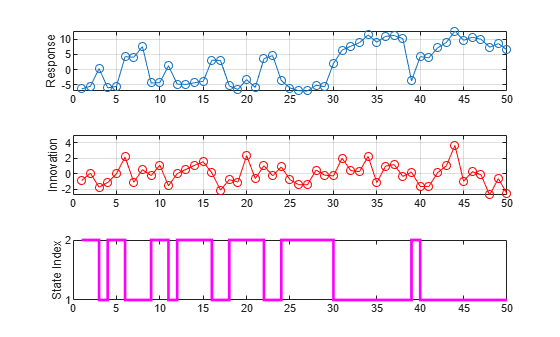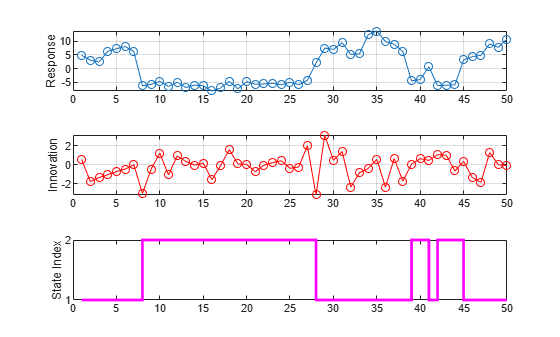# Simulate Univariate Markov-Switching Dynamic Regression Model

This example shows how to generate random response and state paths from a two-state Markov-switching dynamic regression model.

Suppose that an economy switches between two regimes: an expansion and a recession. If the economy is in an expansion, the probability that the expansion persists in the next time step is 0.9, and the probability that it switches to a recession is 0.1. If the economy is in a recession, the probability that the recession persists in the next time step is 0.7, and the probability that it switches to a recession is 0.3.

`$P=\left[\begin{array}{cc}0.9& 0.1\\ 0.3& 0.7\end{array}\right].$`

Also suppose that ${\mathit{y}}_{\mathit{t}}$ is a univariate response process representing an economic measurement that can suggest which state the economy experiences during a period. During an expansion, ${\mathit{y}}_{\mathit{t}}$ is this AR(2) model:

`${y}_{t}=5+0.3{y}_{t-1}+0.2{y}_{t-2}+{\epsilon }_{1t},$`

where ${\epsilon }_{1\mathit{t}}$ is an iid Gaussian process with mean 0 and variance 2. During a recession, ${\mathit{y}}_{\mathit{t}}$ is this AR(1) model:

`${y}_{t}=-5+0.1{y}_{t-1}+{\epsilon }_{2t},$`

where ${\epsilon }_{2\mathit{t}}$ is an iid Gaussian process with mean 0 and variance 1.

### Create Fully Specified Model

Create the Markov-switching dynamic regression model that describes the state of the economy with respect to ${\mathit{y}}_{\mathit{t}}$.

```% Switching mechanism P = [0.9 0.1; 0.3 0.7]; mc = dtmc(P,StateNames=["Expansion" "Recession"]); % AR submodels mdl1 = arima(Constant=5,AR=[0.3 0.2],Variance=2, ... Description="Expansion State"); mdl2 = arima(Constant=-5,AR=0.1,Variance=1, ... Description="Recession State"); % Markov-switching model Mdl = msVAR(mc,[mdl1; mdl2]);```

`Mdl` is a fully specified `msVAR` object.

### Simulate One Path

Simulate one path of responses, innovations, and state indices from the model. Specify a 50-period simulation horizon, that is, a 50-observation path.

```rng("default") [y,e,sp] = simulate(Mdl,50);```

`y`, `e`, and `sp` are 50-by-1 vectors of simulated responses, innovations, and state indices, respectively.

Plot the simulated observations in separate subplots.

```figure tiledlayout(3,1) nexttile plot(y,"o-") ylabel("Response") grid on nexttile plot(e,"ro-") ylabel("Innovation") grid on nexttile stairs(sp,"m",LineWidth=2) ylabel("State Index") yticks([1 2])```### Simulate Multiple Paths

Simulate three separate, independent paths of responses, innovations, and state indices from the model. Specify a 5-period simulation horizon.

`[Y,E,SP] = simulate(Mdl,5,NumPaths=3)`
```Y = 5×3 -5.4315 14.1125 9.6147 -4.1065 12.4008 11.4378 -7.3715 13.4929 -4.1341 1.6877 10.0315 7.0395 2.3238 10.0453 3.3833 ```
```E = 5×3 0.1240 4.1125 -0.3853 1.4367 1.1670 1.5534 -1.9609 1.9502 -0.2779 -0.2796 -1.4965 0.9921 -1.7082 -0.6627 -2.9017 ```
```SP = 5×3 2 1 1 2 1 1 2 1 2 1 1 1 1 1 1 ```
`Mdl.StateNames(SP(2,1))`
```ans = "Recession" ```

`Y`, `E`, and `SP` are 5-by-3 matrices of simulated responses, innovations, and state indices, respectively.

• The simulated response in the second period of the first path is –4.1065.

• The corresponding simulated innovation in the second period of the first path is 1.4367.

• The corresponding simulated state of the economy in the second period of the first path is a recession.

### Simulate Model Containing Regression Component

Include a known regression component in each submodel. During an expansion, the ARX(2) submodel is:

`${y}_{t}=5+0.3{y}_{t-1}+0.2{y}_{t-2}+2{x}_{t}+{\epsilon }_{1t}.$`

During a recession, the ARX(1) submodel is:

`${y}_{t}=-5+0.1{y}_{t-1}+{x}_{t}+{\epsilon }_{2t}.$`

Specify the regression coefficient values of each submodel by setting the `Beta` property using dot notation. Then, create a new Markov-switching model from the modified ARX submodels and the existing Markov chain `mc`.

```mdl1.Beta = 2; mdl2.Beta = 1; Mdl = msVAR(mc,[mdl1; mdl2]);```

`simulate` requires exogenous data over the simulation horizon for the regression component. Generate a 50-by-1 matrix of iid standard normal observations for the required exogenous data.

`x = randn(50,1);`

Simulate one path of responses, innovations, and state indices from the model. Specify a 50-period simulation horizon. Plot the results.

```[y,e,sp] = simulate(Mdl,50,X=x); figure tiledlayout(3,1) nexttile plot(y,"o-") ylabel("Response") grid on nexttile plot(e,"ro-") ylabel("Innovation") grid on nexttile stairs(sp,"m",LineWidth=2) ylabel("State Index") yticks([1 2])```